# 8. Scapegoat Trees

In this chapter, we study a binary search tree data structure, the ScapegoatTree, that keeps itself balanced by partial rebuilding operations. During a partial rebuilding operation, an entire subtree is deconstructed and rebuilt into a perfectly balanced subtree.

There are many ways of rebuilding a subtree rooted at node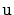into a perfectly balanced tree. One of the simplest is to traverse's subtree, gathering all its nodes into an arrayand then to recursively build a balanced subtree using. If we let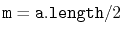, then the element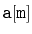becomes the root of the new subtree,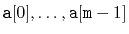get stored recursively in the left subtree and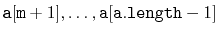get stored recursively in the right subtree.

```    void rebuild(Node<T> u) {
int ns = size(u);
Node<T> p = u.parent;
Node<T>[] a = (Node<T>[]) Array.newInstance(Node.class, ns);
packIntoArray(u, a, 0);
if (p == nil) {
r = buildBalanced(a, 0, ns);
r.parent = nil;
} else if (p.right == u) {
p.right = buildBalanced(a, 0, ns);
p.right.parent = p;
} else {
p.left = buildBalanced(a, 0, ns);
p.left.parent = p;
}
}
int packIntoArray(Node<T> u, Node<T>[] a, int i) {
if (u == nil) {
return i;
}
i = packIntoArray(u.left, a, i);
a[i++] = u;
return packIntoArray(u.right, a, i);
}
Node<T> buildBalanced(Node<T>[] a, int i, int ns) {
if (ns == 0)
return nil;
int m = ns / 2;
a[i + m].left = buildBalanced(a, i, m);
if (a[i + m].left != nil)
a[i + m].left.parent = a[i + m];
a[i + m].right = buildBalanced(a, i + m + 1, ns - m - 1);
if (a[i + m].right != nil)
a[i + m].right.parent = a[i + m];
return a[i + m];
}
```
A call to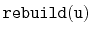takes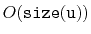time. The subtree built byhas minimum height; there is no tree of smaller height that has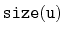nodes.

Subsections

opendatastructures.org